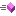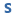﻿ NVector3DD Structure Methods
Nevron .NET Vision
Nevron.GraphicsCore Namespace / NVector3DD Structure

In This Topic
NVector3DD Structure Methods
In This Topic

For a list of all members of this type, see NVector3DD members.

Public Methods
NameDescriptionReturns true if smaller by absolute valueMakes this vector the vector sum of v1 and v2Makes this vector the nozmalized normal of v1, v2 and v3Makes this vector the non nozmalized normal of v1, v2 and v3Overloaded. Computes the cross product of two 3-D vectorsMakes this vector the cross product of vector A and vector BMakes this vector the scalar division of v1 with the specified fDiv factorOverloaded. Computes the dot product of two 3-D vectorsOverloaded. Determines whether this vector is equal to the specified vectorReturns the hash code of this instance.Gets the vector lengthGets the vector length squareInverts the vectorMakes this vector the scalar multiplication of v1 with the specified fMul factorOverloaded. Computes the normalized version of a 3-D vector.Sets the x, y and z coordinates of the vectorStores the maximum values in this vectorMakes this vector the vector sum of v1 and -v2Overriden to provide a string representation of the vector
Top